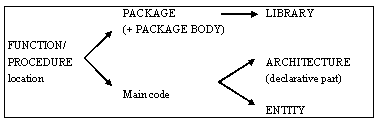Home > VHDL > Advanced VHDL > Function

# Function :

The important application of FUNCTION is to develop new functions for handling the problems such as data type conversions, digital logic operations, arithmetic operations and attributes. By using FUNCTION, the operations can be used in the main code. FUNCTION is similar to a PROCESS in which the statements IF, WAIT, CASE, and LOOP etc. are used. In order to use function in the main code, function body and function call are used.

# Function Location :

The typical locations of a FUNCTION (or PROCEDURE) is shown in figure. The is located in the main code. When placed in a PACKAGE, then a PACKAGE BODY is necessary and should contain the body of each FUNCTION declared in the declarative part of the PACKAGE.

Examples of both cases are presented below.## FUNCTION Located in the Main Code :

Let us consider the positive_edge( ) function of example 1 when it is used in main program itself, the function is located either in the ENTITY or in the declarative part of the ARCHITECTURE.
```LIBRARY ieee;
USE ieee.std_logic_1164.all;
ENTITY dff IS
PORT ( d, clk, rst: IN STD_LOGIC;
q: OUT STD_LOGIC);
END dff;
ARCHITECTURE arch OF dff IS
FUNCTION positive_edge(SIGNAL s: STD_LOGIC)
RETURN BOOLEAN IS
BEGIN
RETURN s'EVENT AND s='1';
END positive_edge;
BEGIN
PROCESS (clk, rst)
BEGIN
IF (rst='1') THEN q <= '0';
ELSIF positive_edge(clk) THEN q <= d;
END IF;
END PROCESS;
END arch;
```

## FUNCTION Located in a PACKAGE :

This example is similar to example above with the only difference being that the FUNCTION located in a PACKAGE can now be reused and shared by other projects. Notice that, when placed in a PACKAGE, the function is indeed declared in the PACKAGE, but described in the PACKAGE BODY.
```LIBRARY ieee;
USE ieee.std_logic_1164.all;
PACKAGE my_package IS
FUNCTION positive_edge(SIGNAL s: STD_LOGIC) RETURN BOOLEAN;
END my_package;
PACKAGE BODY my_package IS
FUNCTION positive_edge(SIGNAL s: STD_LOGIC)
RETURN BOOLEAN IS
BEGIN
RETURN s'EVENT AND s='1';
END positive_edge;
END my_package;
LIBRARY ieee;
USE ieee.std_logic_1164.all;
USE work.my_package.all;
ENTITY dff IS
PORT ( d, clk, rst: IN STD_LOGIC;
q: OUT STD_LOGIC);
END dff;
ARCHITECTURE arch OF dff IS
BEGIN
PROCESS (clk, rst)
BEGIN
IF (rst='1') THEN q <= '0';
ELSIF positive_edge(clk) THEN q <= d;
END IF;
END PROCESS;
END arch;
```